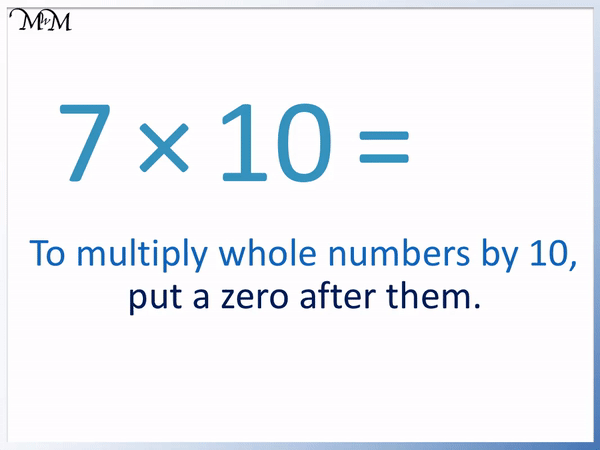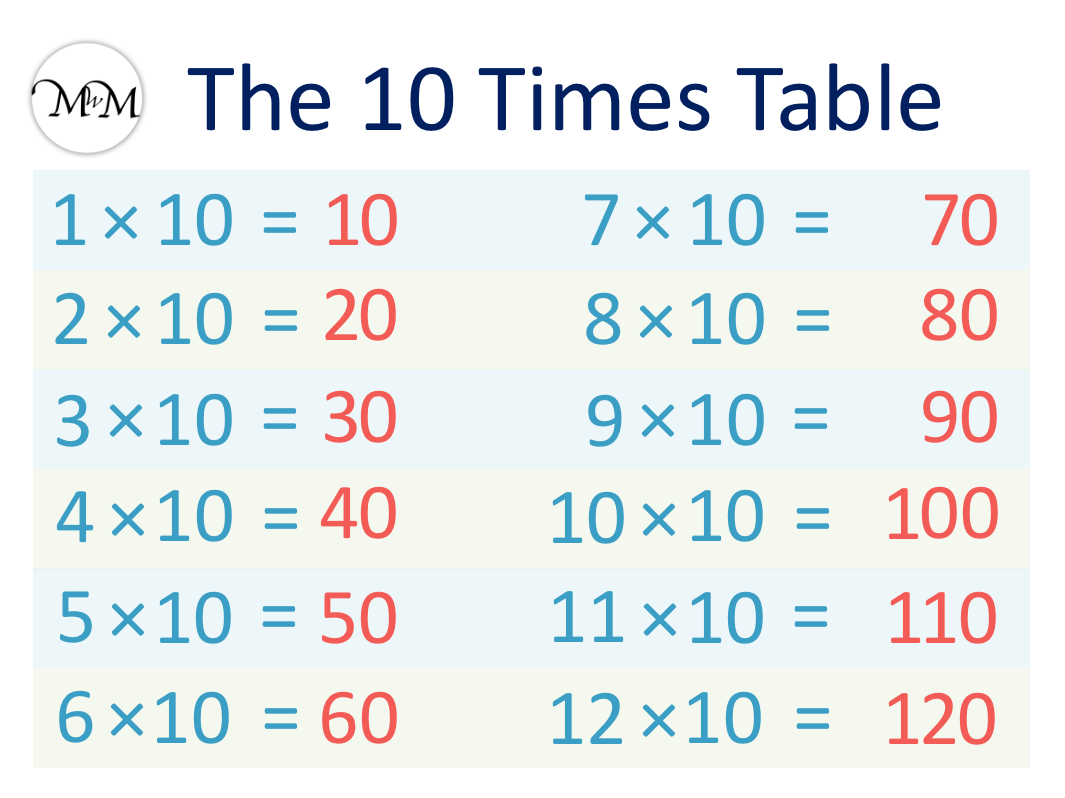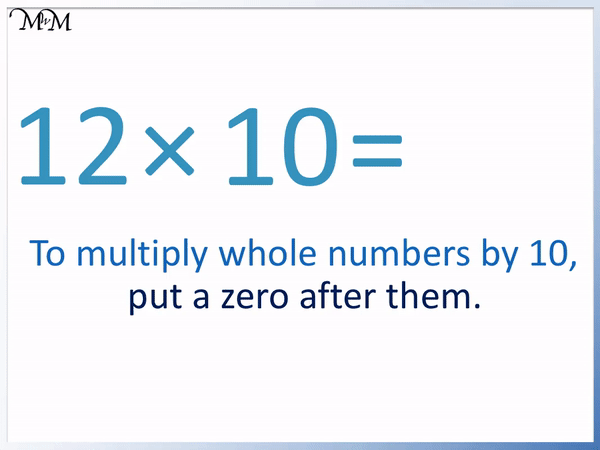# The 10 Times Table

The 10 Times TableThe 10 times table is:

• 1 × 10 = 10
• 2 × 10 = 20.
• 3 × 10 = 30
• 4 × 10 = 40
• 5 × 10 = 50
• 6 × 10 = 60
• 7 × 10 = 70
• 8 × 10 = 80
• 9 × 10 = 90
• 10 × 10 = 100
• 11 × 10 = 110
• 12 × 10 = 120• The 10 times table is made by counting up in tens.
• All numbers in the ten times table end in a zero.
• To multiply a whole number by ten, simply put a zero digit on the end of it.
• This rule makes the ten times table the easiest times table to learn.

All numbers in the 10 times table end in a zero.

To multiply a whole number by ten, simply put a 0 digit after the number being multiplied.• Here is 7 × 10.
• To multiply 7 by 10, we simply write 7 and put a 0 digit after it.
• 7 × 10 = 70.Supporting Lessons#### 10 Times Table Flashcards

Click on the 10 times table flashcards below to memorise the 10 times table.

×
×

=

# The 10 Times Table

## What is the 10 Times Table?

The 10 times table is made by counting up in tens from zero as shown:

• 1 × 10 = 10
• 2 × 10 = 20.
• 3 × 10 = 30
• 4 × 10 = 40
• 5 × 10 = 50
• 6 × 10 = 60
• 7 × 10 = 70
• 8 × 10 = 80
• 9 × 10 = 90
• 10 × 10 = 100
• 11 × 10 = 110
• 12 × 10 = 120

Here is the 10 times table chart showing the complete list of the ten times table.## How to Learn the 10 Times Table

The easiest way to learn the ten times table is to simply put a zero digit after the number being multiplied by 10. This times table trick makes the ten times table the easiest times table to learn. The 10 times table is one of the best times tables to learn first.

We can see the ten times table in the times table chart shown below, with the answers filled in using the ten times table trick.

We simply write the number we are multiplying by 10, followed by a zero digit.The ten times table is easy to learn because it simply involves putting a zero digit on the end of the number being multiplied. Children often find the ten times table easy to remember because it is very similar to the counting numbers of 1, 2, 3, 4, 5, 6, 7, 8, 9, 10, 11 and 12. Instead we have 10, 20, 30, 40, 50, 60, 70, 80, 90, 100, 110 and 120.

## Using the 10 Times Table Trick

The trick to learning the 10 times table is to simply add a 0 to the number being multiplied by 10.

We will look at some examples of using this trick.

Here is 2 × 10.

We are multiplying 2 by 10, so we write the digit ‘2’ and place a ‘0’ digit after it.2 × 10 = 20.

Here is 7 ×10.

We are multiplying 7 by 10, so we write the digit ‘7’ and place a ‘0’ digit after it.7 ×10 = 70.

This trick works with any whole number no matter how large.

Here we have 12 × 10.

We simply write ’12’ with a ‘0’ digit afterwards.12 × 10 = 120.

This rule only works for whole numbers but it makes the ten times table a very simple times table to start learning.Now try our lesson on Multiplying by 10 where we learn how to multiply any number by 10.error: Content is protected !!